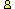CTK Exchange

 Subject: "Another solution to geoprobability problem" Previous Topic | Next Topic
 ConferencesThe CTK ExchangeGuest bookTopic #1289Printer-friendly copyEmail this topic to a friend Reading Topic #1289
Prasanna Medaguest
Jul-23-06, 06:39 AM (EST)

"Another solution to geoprobability problem"

 The solution https://www.cut-the-knot.org/triangle/geoprobability.shtml uses the bary centric co-ordinates. I think it is easy to map the possible areas and find the probability.The total possible points that satisy the conditions are 0<=x<<1, 0<=y<=1, 0<=z<=1 and x + y + z = 1 is a traingle on the plane x+y+z =1 with x-y plane, y-z plane and x-z plane as sides. Its three vertices are (1,0,0),(0,0,1) and (0,1,0). Its side is sqrt(2) units. Its area is sqrt(3)/4*(sqrt(2)*sqrt(2))The total possible points that satisfy the conditions 0<=x<=1/2, 0<=y<=1/2, 0<=z<=1/2, x + y + z = 1 is another trinagle with sides (1/2,1/2,0), (0,1/2,1/2) and (1/2,0,1/2). Its side is sqrt(2)/2 units and its area is sqrt(3)/4*sqrt(2)/2*sqrt(2)/2. This trinagle is actually formed with mid points of the sides of the above traingle.The probability is thus 1/4, the ratio of areas of the traingles.

Alert | IP Printer-friendly page | Reply | Reply With Quote | Top
alexbCharter Member
1865 posts
Jul-24-06, 03:40 PM (EST)1. "RE: Another solution to geoprobability problem"
In response to message #0

 I would not call this "another solution". The difference between what you are saying and the page you refer to is two-fold: You observe the fact that the barycentric coordinates are nothing but the regular Cartesian coordinates in the plane x + y + z = 1. This is a very good observation. You explicitly compute the areas of the two triangles involved. But there is no need for that. As a medial triangle, the small one has 1/4 of the area of the big triangle.

Alert | IP Printer-friendly page | Reply | Reply With Quote | Top

 Conferences | Forums | Topics | Previous Topic | Next Topic
 Select another forum or conference Lobby The CTK Exchange (Conference)   |--Early math (Public)   |--Middle school (Public)   |--High school (Public)   |--College math (Public)   |--This and that (Public)   |--Guest book (Public) Educational Press (Conference)   |--No Child Left Behind (Public)   |--Math Wars (Public)   |--Mathematics and general education (Public)You may be curious to visit the old Guest book.
Please do not post there.